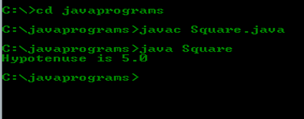## Write a program which demonstrate dynamic initialization.

Category : Java | Sub Category : Data type, Variable and Arays | By Runner Dev Last updated: 2020-09-28 14:57:00 Viewed : 191

## Write a program which demonstrate dynamic initialization.

DESCRIPTION: This program computes the length of the hypotenuse of right triangle given the length of two opposing sides.

Source Code:

/**

*

* Write a program which demonstrate dynamic initialization.

*/

class Square { // This line daclare a class "Square" and the class is start from here .

public static void main(String a[]) { // This line begins the main() method.

double x = 3.0, y = 4.0; // Initiallize two double variables.

double z = Math.sqrt(x * x + y * y); // z is initialized and declare calue of z

System.out.println("Hypotenuse is " + z); // this line print value of Hypotenus

} // end main funcation

}// end of class name

OUTPUT: